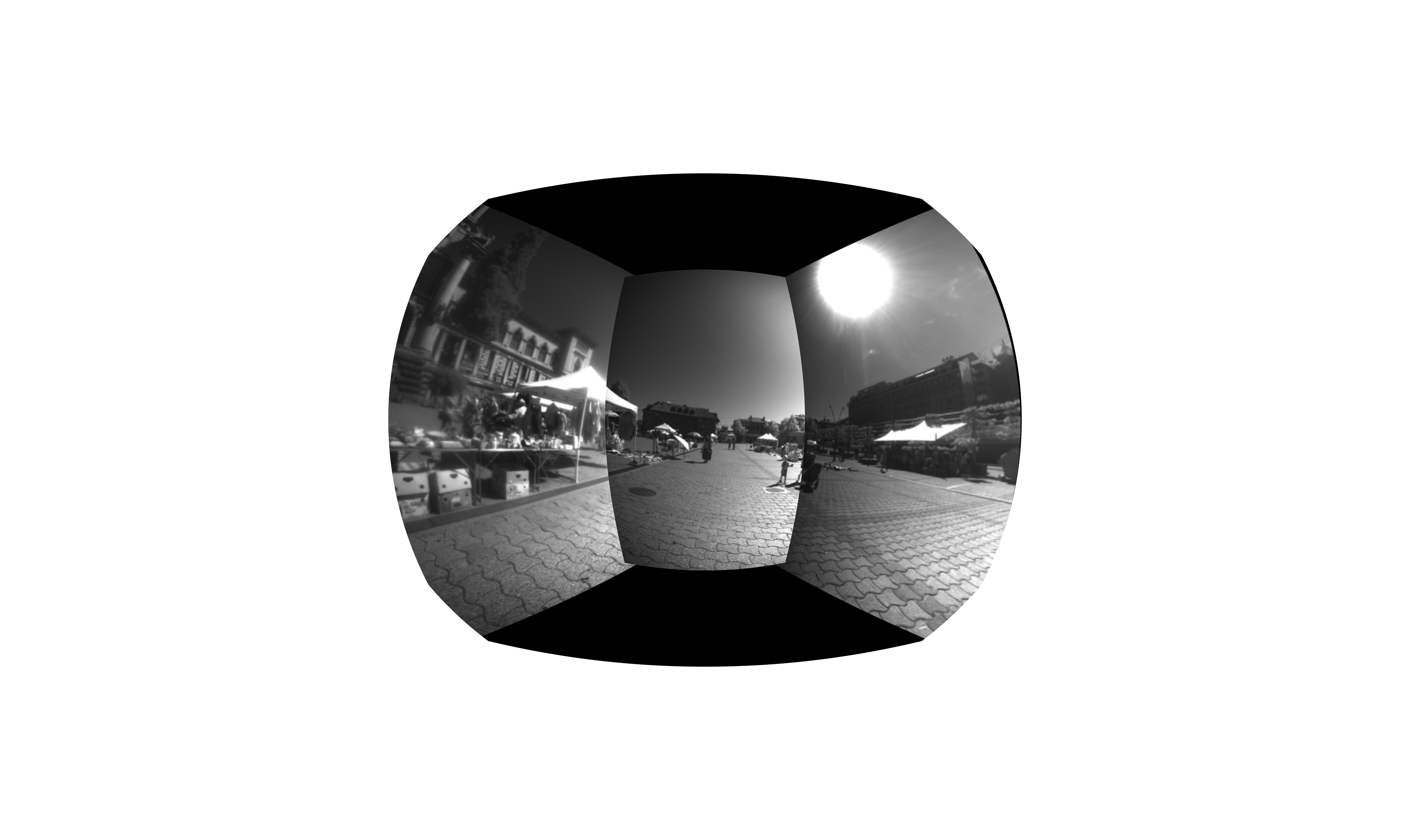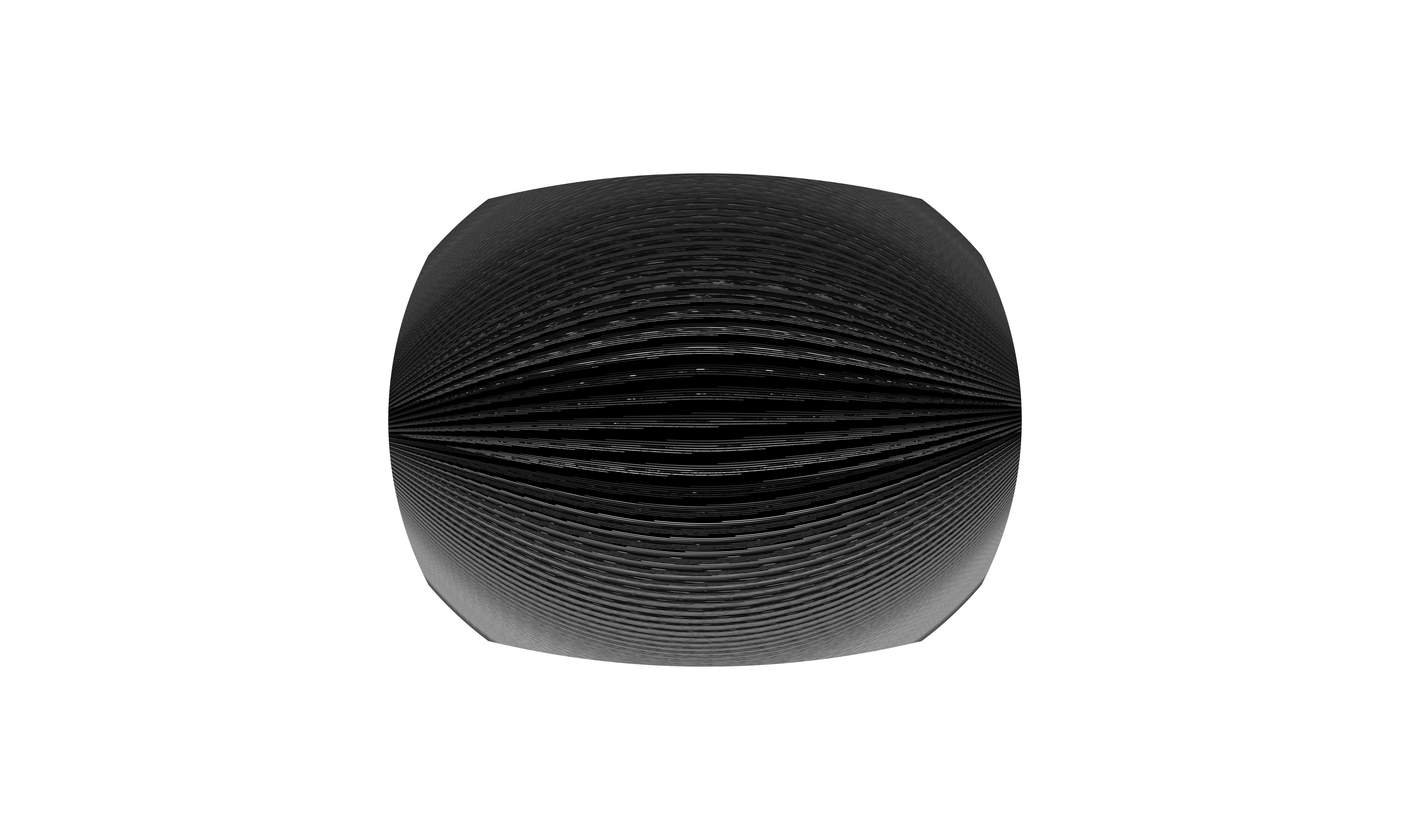/ wand Public

# Image.from_array() fails on one of two seemingly identical arrays#583

Closed
opened this issue Jul 19, 2022 · 1 comment
Closed

# Image.from_array() fails on one of two seemingly identical arrays #583

opened this issue Jul 19, 2022 · 1 comment
Labels
Milestone

### nek11 commented Jul 19, 2022 • edited

 I have this notebook: ``````import cv2 import numpy as np import matplotlib.pyplot as plt import glob from wand.image import Image class BipedStitcher: `````` ``````def _init_(self): self._width = 270 self._height = 480 M_01 = np.array([[ 9.73331494e-01, -8.01939095e-01, 2.23343892e+01], [ 2.72445372e-02, 5.90069057e-02, 6.44154273e+02], [ 2.28304381e-05, -1.25424366e-03, 1.00000000e+00]]) M_21 = np.array([[ 1.58019986e+01, 1.35065361e+01, -9.44831124e+03], [-8.03741771e-02, 1.67267572e+01, -1.08396169e+04], [-3.94436455e-05, 2.12285957e-02, 1.00000000e+00]]) S = np.array([ [self._width / 720, 0, 0], [0, self._width / 720, 0], [0, 0, 1] ]) self._matrix_0 = S.dot(M_01.dot(np.linalg.inv(S))) self._matrix_2 = S.dot(M_21.dot(np.linalg.inv(S))) K = np.array([ [239.417, 0.0, 239.291], [0.0, 239.417, 128.642], [0.0, 0.0, 1.0] ]) num, R, T, N = cv2.decomposeHomographyMat(M_01, K) self._canvas_w, self._canvas_h = 10 * self._width, 10 * self._height x_offset = self._canvas_w // 2 - self._height // 2 y_offset = self._canvas_h // 2 self._translation_matrix = np.array([ # translation matrix [1,0,x_offset], [0,1,y_offset], [0,0,1] ], dtype=np.float32) def _overlay_images(self, left_warped, middle, right_warped): # Select all non-black pixels left_mask = np.float32(np.any(left_warped != [0, 0, 0], axis=-1)) middle_mask = np.float32(np.any(middle != [0, 0, 0], axis=-1)) right_mask = np.float32(np.any(right_warped != [0, 0, 0], axis=-1)) # XOR mask in the middle xor_mask_left_mid = cv2.bitwise_xor(left_mask, middle_mask) xor_mask_final = cv2.bitwise_xor(xor_mask_left_mid, right_mask).astype(int) final_mask = np.stack([xor_mask_final, xor_mask_final, xor_mask_final], axis=2) # Stitch the three images stitched_image = left_warped*final_mask + right_warped*final_mask + middle stitched_image = np.rot90(stitched_image, k=1) return stitched_image def _undistort_image(self, stitched_image): # Cut image to center it and transform to int for wand stitched_image_cut = (stitched_image[:, 270:, :]).astype(np.uint8) cv2.imwrite("temp_save.png", stitched_image_cut) array = cv2.imread("temp_save.png") print(np.array_equal(stitched_image_cut, array)) with Image.from_array(np.copy(array)) as wand_image: wand_image.virtual_pixel = 'transparent' wand_image.distort('barrel_inverse', (0.0, 0.0, -2.5, 4.5)) wand_image.distort('barrel', (1, 0.0, 0.0, 1.5)) undistorted_image = np.array(wand_image) cv2.imwrite("loaded_from_cv2.png", undistorted_image) with Image.from_array(stitched_image_cut) as wand_image_2: wand_image_2.virtual_pixel = 'transparent' wand_image_2.distort('barrel_inverse', (0.0, 0.0, -2.5, 4.5)) wand_image_2.distort('barrel', (1, 0.0, 0.0, 1.5)) undistorted_image_2 = np.array(wand_image_2) cv2.imwrite("loaded_from_array.png", undistorted_image_2) return undistorted_image def run(self, left_image, middle_image, right_image): warped_img_right = cv2.warpPerspective(right_image, self._translation_matrix.dot(self._matrix_0), dsize=(self._canvas_w, self._canvas_h), borderMode=cv2.BORDER_CONSTANT, borderValue=(0,0,0)) translated_img_middle = cv2.warpPerspective(middle_image, self._translation_matrix, dsize=(self._canvas_w, self._canvas_h), borderMode=cv2.BORDER_CONSTANT, borderValue=(0,0,0)) warped_img_left = cv2.warpPerspective(left_image, self._translation_matrix.dot(self._matrix_2), dsize=(self._canvas_w, self._canvas_h), borderMode=cv2.BORDER_CONSTANT, borderValue=(0,0,0)) stitched_image = self._overlay_images(warped_img_left, translated_img_middle, warped_img_right) undistorted_image = self._undistort_image(stitched_image) `````` ``````left_image = cv2.imread("test/left.jpg", cv2.COLOR_RGB2GRAY) middle_image = cv2.imread("test/middle.jpg", cv2.COLOR_RGB2GRAY) right_image = cv2.imread("test/right.jpg", cv2.COLOR_RGB2GRAY) stitcher = BipedStitcher() stitcher.run(left_image, middle_image, right_image) `````` For which you will need the zip file I have attached. test.zip Now, a very weird output is produced: In line 65, I save an array called "stitched_image_cut" with cv2 as "temp_save.png", then load it again with cv2 just after (named "array"), and test that both arrays are equal, which they are. Then, I use the "Image.from_array" function on the loaded from cv2 array "array", apply some transformations and save it as "loaded_from_cv2.png". This is the result:Just after, I do the same thing but this time with "stitched_image_cut", which is supposed to be exactly the same array as "array". This time, this is what I get:Can anyone tell me what in the world is going on? The text was updated successfully, but these errors were encountered:

### emcconville commented Jul 19, 2022 • edited

 Looks like a bug with stride handling. Although the ultimate data is the same, one data-buffer is laid out in memory with `K` order, and the other as 'C' order. Short fix Just set the order when copying data (`np.copy(img, order='C')`) ```... with Image.from_array(np.copy(array, order='C')) as wand_image: ... with Image.from_array(np.copy(stitched_image_cut, order='C')) as wand_image_2:```